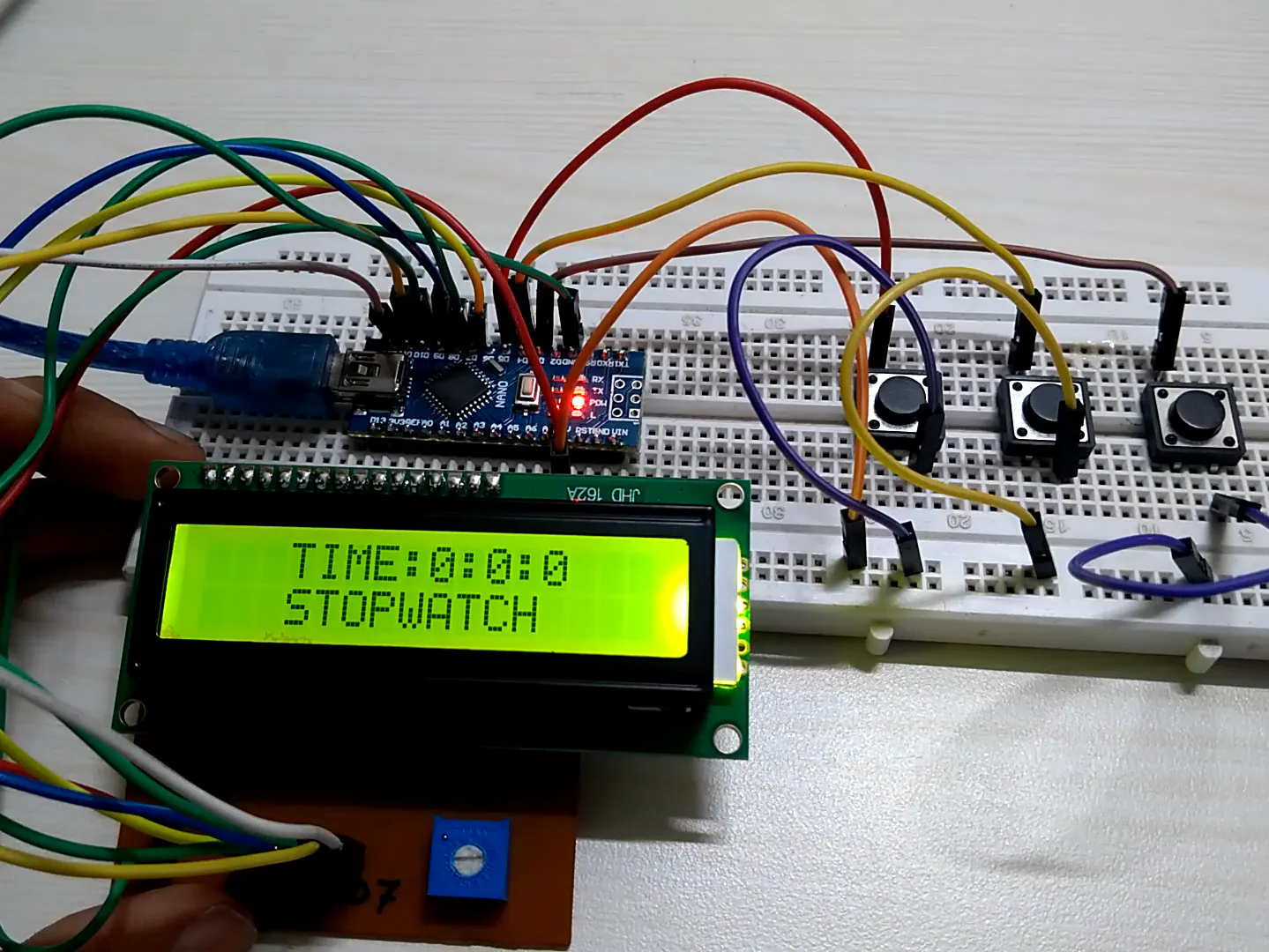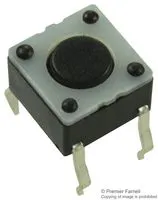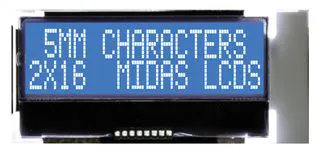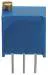# Make Your Own Stopwatch With Arduino

By using an Arduino board, switches and an LCD display create a simple stopwatch

BeginnerShowcase (no instructions)305## Things used in this project

### Hardware components×1Tactile Switch, Top Actuated
×3Alphanumeric LCD, 16 x 2
×1Resistor 1k ohm
×1Multi-Turn Precision Potentiometer- 10k ohms (25 Turn)
×1Arduino Nano R3
×1Jumper wires (generic)
×1

## Code

### StopWatch

C/C++
```/*Simple LCD stopwatch program with stop, start, reset and lap buttons.*/

//including liblary for LCD
#include <LiquidCrystal.h>

//setting up LCD INPUT pins
LiquidCrystal lcd(12,11,10,9,8,7);// (RS,E D4,D5,D6,D7)

//setting hours, minutes, secound and miliseconds to 0
int h=0;
int m=0;
int s=0;
int ms=0;

//defines pin for all buttons
const int startPin = 3;
const int stopPin = 4;
const int resetPin = 5;

//defines starting points
int start=0;
int stop1=0;
int reset=0;

void stopwatch()
{

lcd.setCursor(3,0);   //setting start point on lcd
lcd.print("TIME:");   //display TIME
lcd.print(h);         //display hours
lcd.print(":");
lcd.print(m);         //display minutes
lcd.print(":");
lcd.print(s);         //display seconds
lcd.setCursor(3,1);
lcd.print("         ");

ms=ms+10;
delay(10);

if(ms==590)
{

ms=0;
s=s+1;
}

if(s==60)     //if state for counting up minutes
{
s=0;
m=m+1;
}

if(m==60)      //if state for counting up hours
{
m=00;
h=h+01;
}

if(stop1 == HIGH)    //checking if button is pressed
{
stopwatch_stop();   //calls the stopwatch_stop function
}
else
{
stopwatch();    //calls the stopwatch function
}
}

void stopwatch_stop()
{
lcd.setCursor(3,0);
lcd.print("TIME:");
lcd.print(h);
lcd.print(":");
lcd.print(m);
lcd.print(":");
lcd.print(s);
lcd.setCursor(3,1);
lcd.print("         ");

if(start == HIGH)
{
stopwatch();    //calls the stopwatch function
}

if(reset == HIGH)
{
stopwatch_reset();    //calls the stopwatch_reset function
loop();
}
if(reset == LOW)
{
stopwatch_stop();    //calls the stopwatch_stop function
}
}

void stopwatch_reset()
{
lcd.clear();
h=00;    //seting hours to 0
m=00;    //seting minutes to 0
s=00;    //seting seconds to 0
return;  //exiting the program and returning to the point where entered the program
}

void setup()
{

lcd.begin(16 ,2);  //starting LCD

//defining pins if they are INPUT or OUTPUT pins
pinMode(startPin, INPUT);
pinMode(stopPin, INPUT);
pinMode(resetPin, INPUT);

}
void loop()
{

lcd.setCursor(3,1);
lcd.print("STOPWATCH");
lcd.setCursor(3,0);
lcd.print("TIME:");
lcd.print(h);
lcd.print(":");
lcd.print(m);
lcd.print(":");
lcd.print(s);

if(start == HIGH)
{
stopwatch();  //calls the stopwatch function
}
}

```

## Credits

### nimishac

3 projects • 0 followers